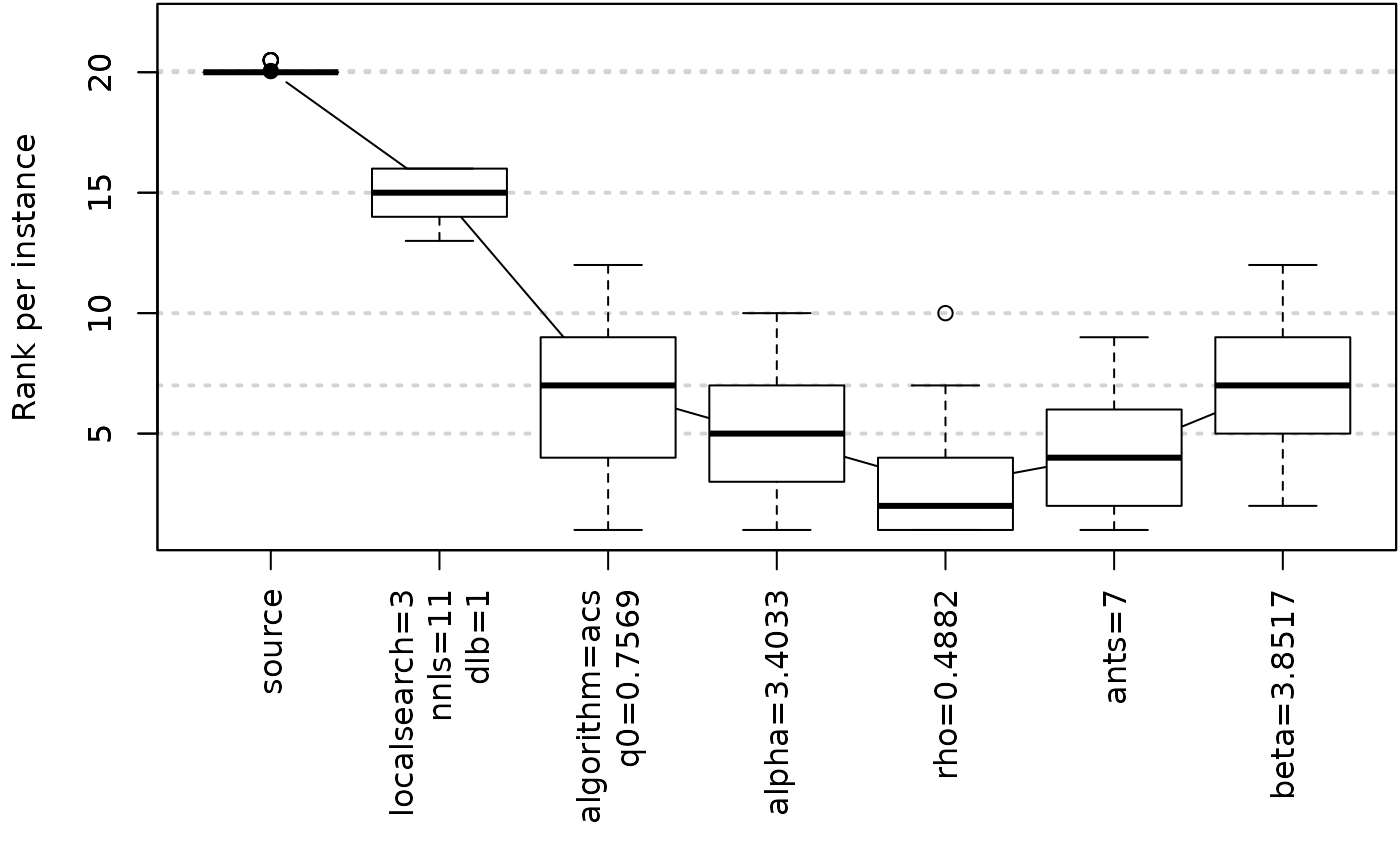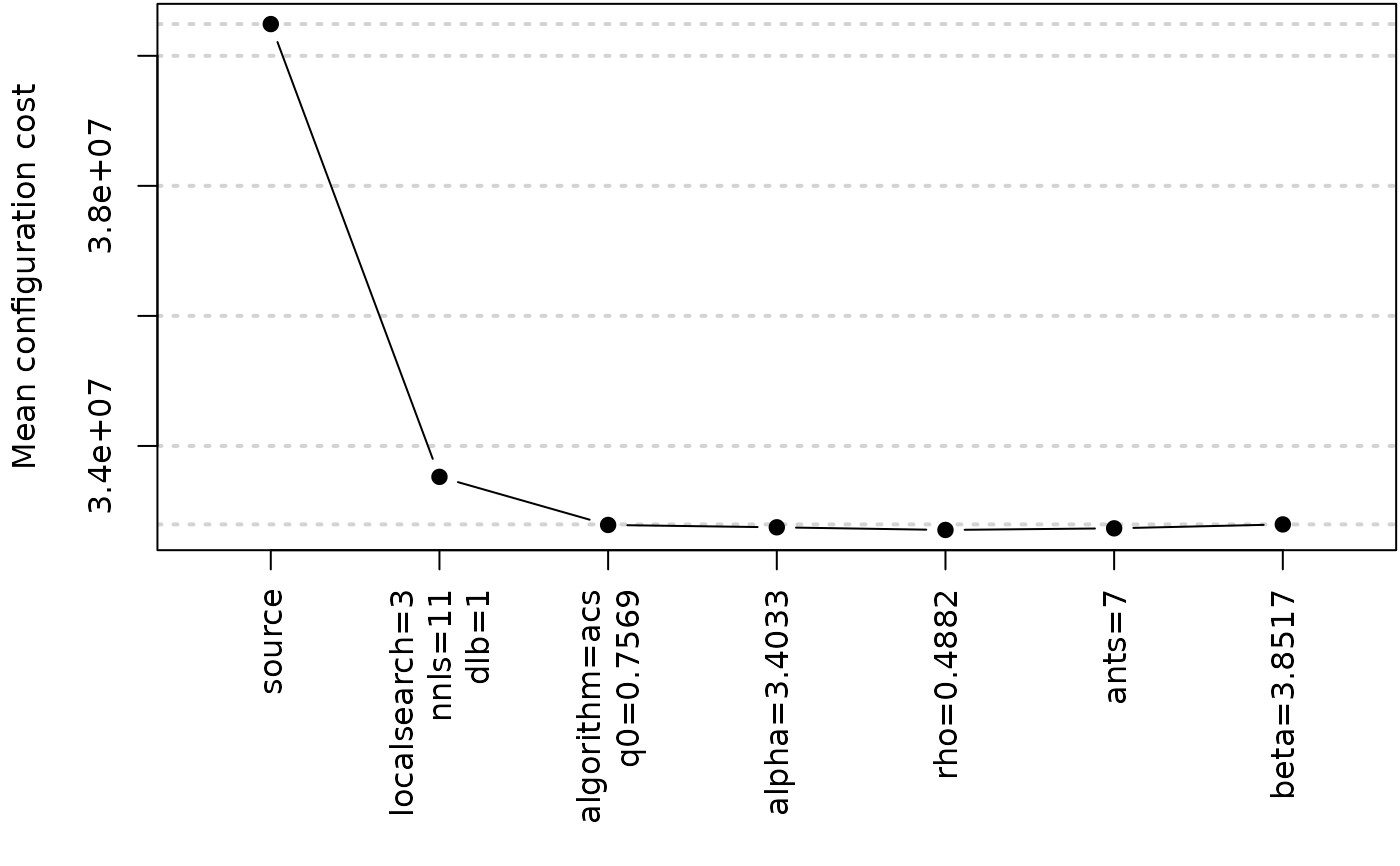Create plot from an ablation log

## Usage

plotAblation(
ablog,
pdf.file = NULL,
pdf.width = 20,
type = c("mean", "boxplot", "rank"),
n = 0L,
mar = par("mar"),
ylab = "Mean configuration cost",
ylim = NULL,
...
)

## Arguments

ablog

(list()|character(1)) Ablation log object returned by ablation(). Alternatively, the path to an .Rdata file, e.g., "log-ablation.Rdata", from which the object will be loaded.

pdf.file

Output filename.

pdf.width

Width provided to create the pdf file.

type

Type of plot. Supported values are "mean" and "boxplot". Adding "rank" will plot rank per instance instead of raw cost value.

n

(integer(1)) Number of parameters included in the plot. By default all parameters are included.

mar

Vector with the margins for the ablation plot.

ylab

Label of y-axis.

ylim

Numeric vector of length 2 giving the y-axis range.

...

Further graphical parameters may also be supplied as arguments. See graphics::plot.default().

ablation() ablation_cmdline()

## Author

Leslie Pérez Cáceres and Manuel López-Ibáñez

## Examples

logfile <- file.path(system.file(package="irace"), "exdata", "log-ablation.Rdata")
plotAblation(ablog = logfile)plotAblation(ablog = logfile, type = "mean")plotAblation(ablog = logfile, type = c("rank","boxplot"))